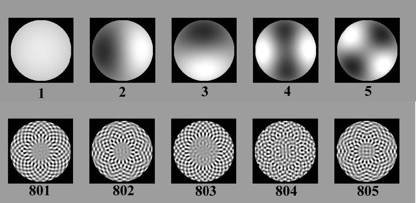# Axial Tomography by filtered least squares

#### The EigenfunctionsFig 3: Each of the eigenvectors of HTH (one of the the columns of V) may be mapped onto the lattice since each component of the vector is associated with a lattice point. When we do this we generate two-dimensional functions in the circular space we have chosen for the solution. These functions are (by definition) orthonormal. Because we have chosen a circular boundary, they look rather like cylinder functions. In fact they have a symmetry characteristic of the geometry of the problem. © Kenneth C. Holmes

Each of the eigenvectors of HTH (one of the the columns of V) may be mapped onto the lattice since each component of the vector is associated with a lattice point. When we do this we generate two-dimensional functions in the circular space we have chosen for the solution. These functions are (by definition) orthonormal. Because we have chosen a circular boundary, they look rather like cylinder functions. In fact they have a symmetry characteristic of the geometry of the problem. Some examples from a case considered below are shown in Fig 3.

Zur Redakteursansicht